Скачать презентацию General Formulation — A Turbojet Engine Relevant physical

bf93f97eee0ea67a9f7a27e57c8ba107.ppt

• Количество слайдов: 11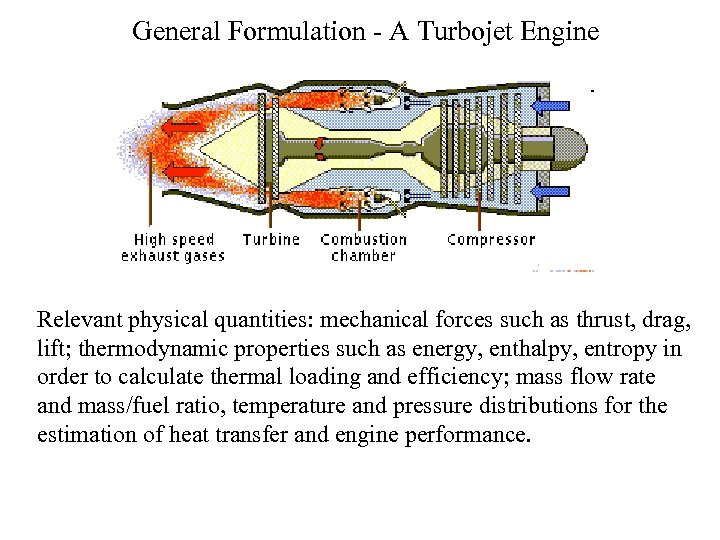General Formulation - A Turbojet Engine Relevant physical quantities: mechanical forces such as thrust, drag, lift; thermodynamic properties such as energy, enthalpy, entropy in order to calculate thermal loading and efficiency; mass flow rate and mass/fuel ratio, temperature and pressure distributions for the estimation of heat transfer and engine performance.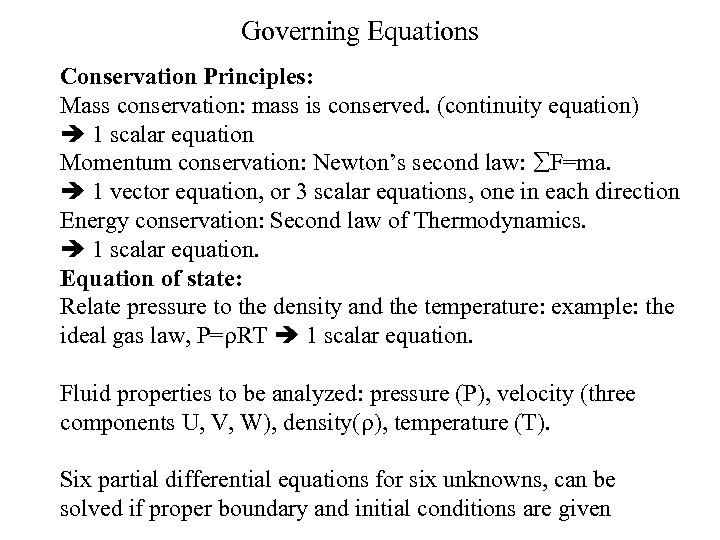Governing Equations Conservation Principles: Mass conservation: mass is conserved. (continuity equation) 1 scalar equation Momentum conservation: Newton’s second law: F=ma. 1 vector equation, or 3 scalar equations, one in each direction Energy conservation: Second law of Thermodynamics. 1 scalar equation. Equation of state: Relate pressure to the density and the temperature: example: the ideal gas law, P=r. RT 1 scalar equation. Fluid properties to be analyzed: pressure (P), velocity (three components U, V, W), density(r), temperature (T). Six partial differential equations for six unknowns, can be solved if proper boundary and initial conditions are given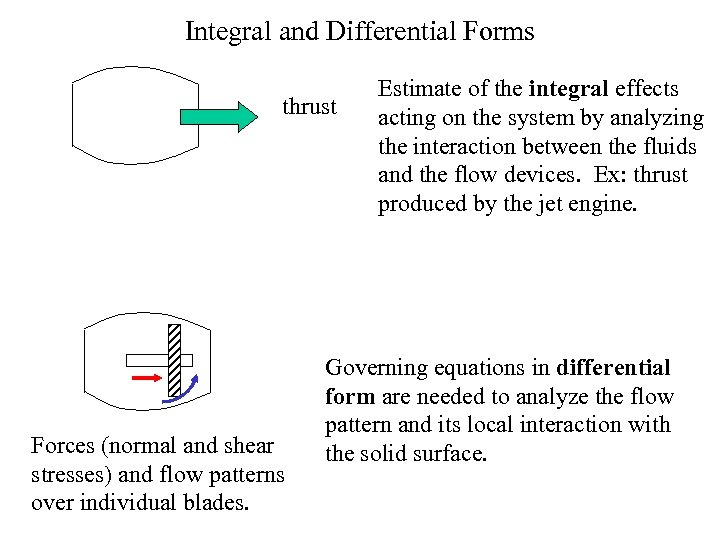Integral and Differential Forms thrust Forces (normal and shear stresses) and flow patterns over individual blades. Estimate of the integral effects acting on the system by analyzing the interaction between the fluids and the flow devices. Ex: thrust produced by the jet engine. Governing equations in differential form are needed to analyze the flow pattern and its local interaction with the solid surface.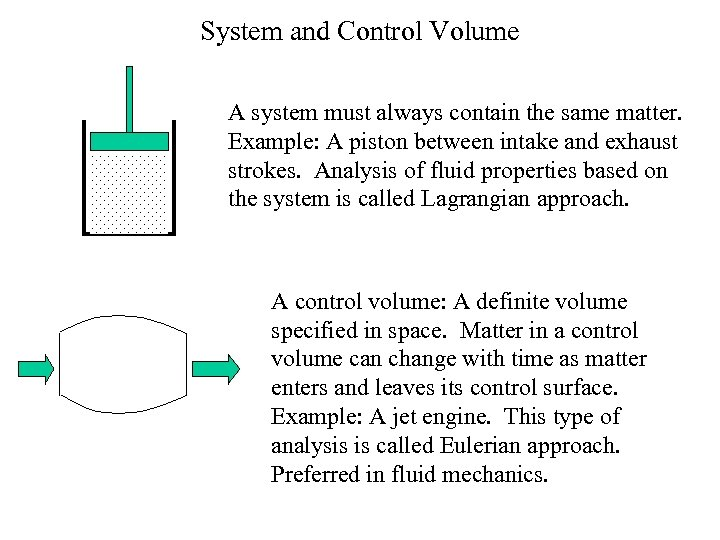System and Control Volume A system must always contain the same matter. Example: A piston between intake and exhaust strokes. Analysis of fluid properties based on the system is called Lagrangian approach. A control volume: A definite volume specified in space. Matter in a control volume can change with time as matter enters and leaves its control surface. Example: A jet engine. This type of analysis is called Eulerian approach. Preferred in fluid mechanics.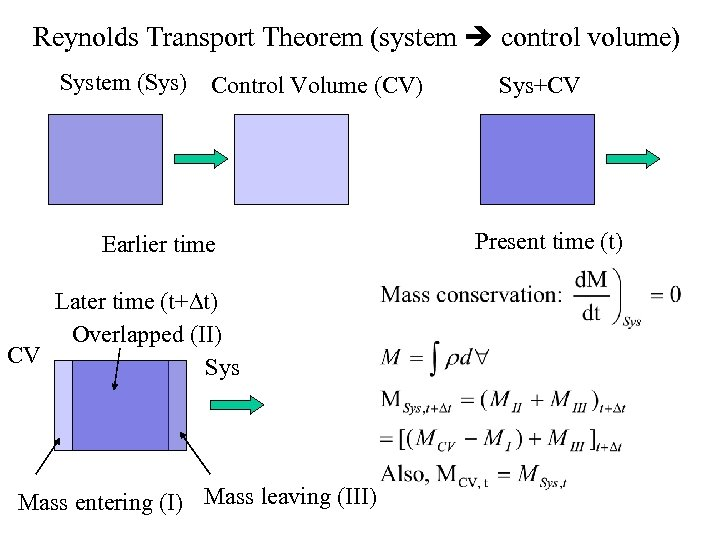Reynolds Transport Theorem (system control volume) System (Sys) Control Volume (CV) Earlier time Later time (t+Dt) Overlapped (II) CV Sys Mass entering (I) Mass leaving (III) Sys+CV Present time (t)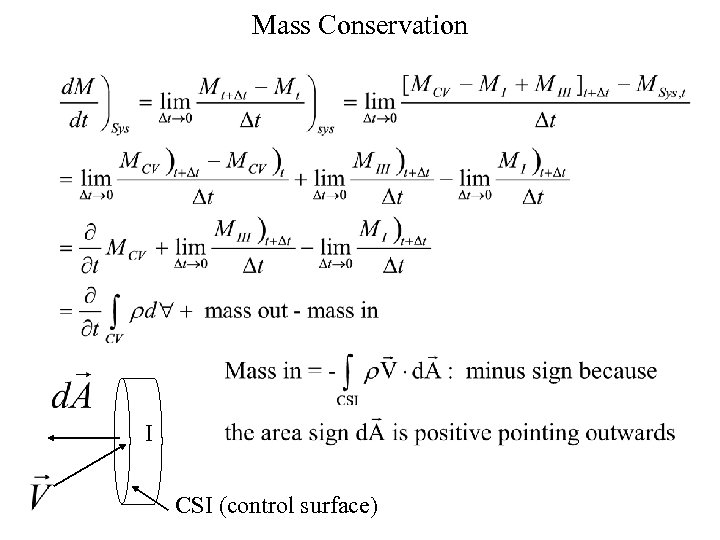Mass Conservation I CSI (control surface)Mass Conservation III CSIII (control surface) Mass Conservation in control volume form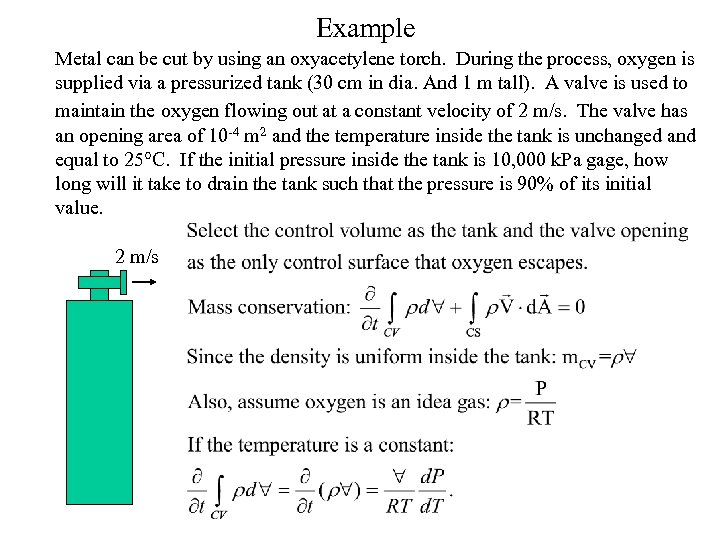Example Metal can be cut by using an oxyacetylene torch. During the process, oxygen is supplied via a pressurized tank (30 cm in dia. And 1 m tall). A valve is used to maintain the oxygen flowing out at a constant velocity of 2 m/s. The valve has an opening area of 10 -4 m 2 and the temperature inside the tank is unchanged and equal to 25°C. If the initial pressure inside the tank is 10, 000 k. Pa gage, how long will it take to drain the tank such that the pressure is 90% of its initial value. 2 m/s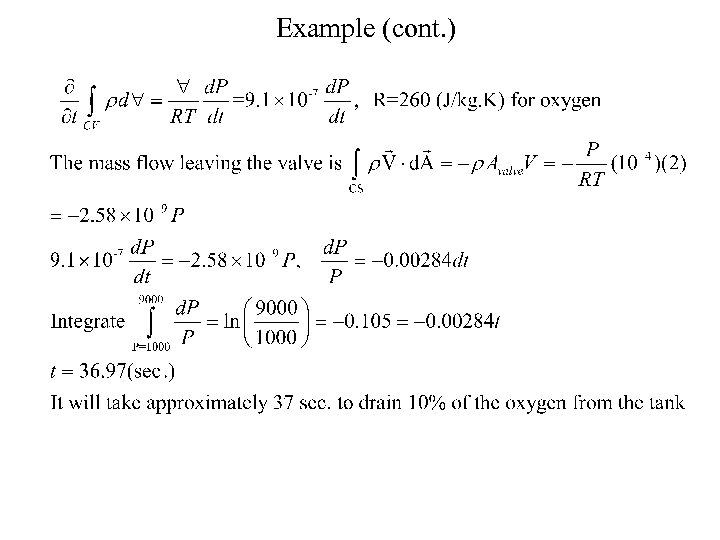Example (cont. )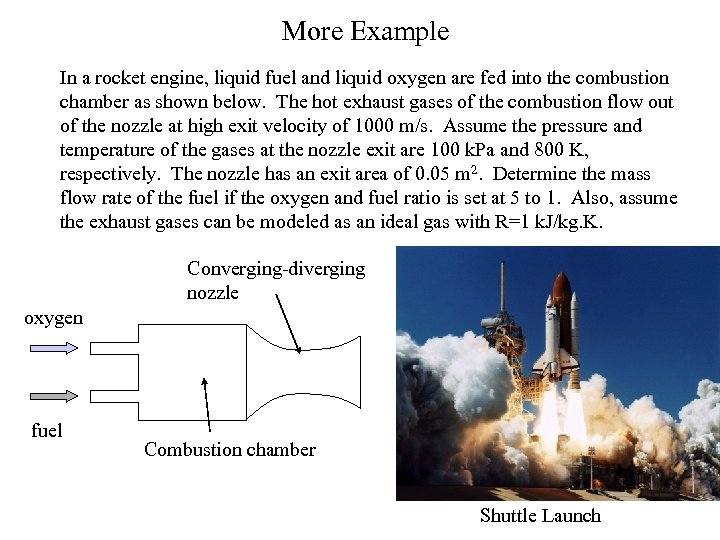More Example In a rocket engine, liquid fuel and liquid oxygen are fed into the combustion chamber as shown below. The hot exhaust gases of the combustion flow out of the nozzle at high exit velocity of 1000 m/s. Assume the pressure and temperature of the gases at the nozzle exit are 100 k. Pa and 800 K, respectively. The nozzle has an exit area of 0. 05 m 2. Determine the mass flow rate of the fuel if the oxygen and fuel ratio is set at 5 to 1. Also, assume the exhaust gases can be modeled as an ideal gas with R=1 k. J/kg. K. Converging-diverging nozzle oxygen fuel Combustion chamber Shuttle LaunchExample (cont. )